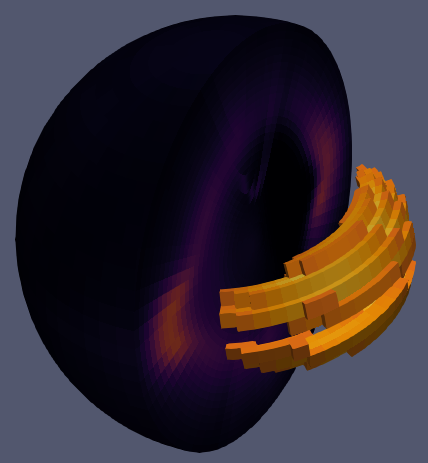# plotting TIE-GCM netcdf output

Hi all,

I am new to Para View, and this forum. I have already done the first quarter of the tutorial and tried to find some answers in the Internet but I was not very successful. I am using Para View 5.8.0 on Manjaro Kyria 19.0.2.

I want to plot the output of the NCAR TIE-GCM (Thermosphere-Ionosphere-Electrodynamics General Circulation Model).

The output is saved in netCDF files following the CF-Conventions (CF-1.0). The fields are given on spherical coordinates: latitude, longitude and pressure scale height as vertical component. When choosing 5 degree resolution the netcdf file has the following entries (extract):

``````dimensions:
time = UNLIMITED;   // (4 currently)
lon = 72;
lat = 36;
ilev = 29;
variables:
double time(time=4);
:long_name = "time";
:units = "minutes since 2004-3-20 0:0:0";
:initial_year = 2004; // int
:initial_day = 80; // int
:initial_mtime = 80, 0, 0; // int
double lon(lon=72);
:long_name = "geographic longitude (-west, +east)";
:units = "degrees_east";
:standard_name = "longitude";
double lat(lat=36);
:long_name = "geographic latitude (-south, +north)";
:units = "degrees_north";
:standard_name = "latitude";
double ilev(ilev=29);
:long_name = "interface levels";
:short_name = "ln(p0/p)";
:units = "";
:positive = "up";
:standard_name = "atmosphere_ln_pressure_coordinate";
:formula_terms = "p0: p0 lev: ilev";
:formula = "p(k) = p0 * exp(-ilev(k))";
:computed_standard_name = "air_pressure";
float NE(time=4, ilev=29, lat=36, lon=72);
:long_name = "ELECTRON DENSITY";
:units = "cm-3";
:missing_value = 1.0E36; // double
float ZG(time=4, ilev=29, lat=36, lon=72);
:long_name = "Geometric Height ZG";
:units = "cm";
:missing_value = 1.0E36; // double
``````

I have successfully opened a corresponding dataset using the ‘NetCDF Reader’, but I have some questions

1. Representation
If I choose ‘outline’ the boundary is a semicircle. I would expect a sphere

When changing the representation to ‘points’, only a slice of all internal points is shown. Why are not all points plotted?

However, surface plots are working as expected2. Vertical Coordinate
I would like to use the geometric height (field ZG ) as vertical coordinate instead of the pressure scale height. There is the filter ‘Warp by Vector’ which probably can do this. But ZG is a 4D scalar field and not a vector field. Of course I can compute the cartesian coordinates from ZG, latitude and longitude, and include them in the netcdf file, but Para View can not open 5D variables (lat,lon,time,ilev,dim vector). I get the error message:

`````` ERROR: In /build/paraview/src/ParaView-v5.8.0/VTK/IO/NetCDF/vtkNetCDFReader.cxx, line 761
vtkNetCDFCFReader (0x55cbbdb74b30): More than 3 dims + time not supported in variable
``````

I have tried ‘Warp by Scalar’ but all points are then shifted a long the the same axis.

So is there a way to use the geometric coordinates using Para View?

1. Contour
After applying the ‘Cell Data to Point Data Filter’ it is possible to use the contour filter.
But I get the following error message:

`````` Generic Warning: In /build/paraview/src/ParaView-v5.8.0/VTK/Common/Core/vtkMath.cxx, line 1314
Unable to factor linear system
Generic Warning: In /build/paraview/src/ParaView-v5.8.0/VTK/Filters/Core/vtkGridSynchronizedTemplates3D.cxx, line 295
``````

Are there other ways to solve these problems, e.g., converting the netcdf file to a different format?

Thanks a lot for your help!

Sorry, I forget to include the file. You can download it here:
https://uni-bonn.sciebo.de/s/WBBtavjODIDnKoe

If I choose ‘outline’ the boundary is a semicircle. I would expect a sphere

This is how curvilinear grid outline is represented in ParaView.

When changing the representation to ‘points’, only a slice of all internal points is shown. Why are not all points plotted?

Only the point on the “surface” are reoresented, it is kinda breaking up for curvilinear grid though.

But ZG is a 4D scalar field and not a vector field.

Use calculator filter to create a 3D vector.

After applying the ‘Cell Data to Point Data Filter’ it is possible to use the contour filter. But I get the following error message:

Looks like a bug with curvilinear grid and contour filter. The results still seems correct though.

In any case, you can convert your dataset to unstructured grid by using a AppendDataset filter.

I explain my pipeline here in more detail for other potential users:

2. Apply the `Append Datasets` Filter to use unstructured grid representation
3. Apply the `Cell Data to Point Data` Filter
4. Apply the `Calculator` Filter to compute a height vector
`ZG/100000*norm(coords)`
5. Apply the `Warp by Vector` Filter